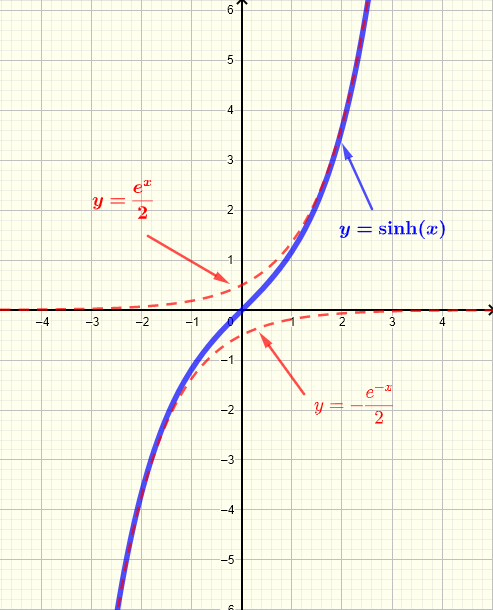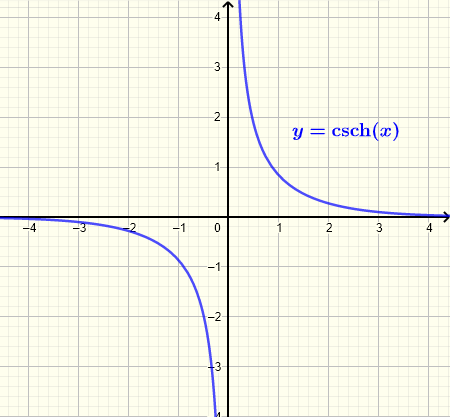# Graphs of Hyperbolic Functions

The graphs and properties such as domain, range and asymptotes of the 6 hyperbolic functions: sinh(x), cosh(x), tanh(x), coth(x), sech(x) and csch(x) are presented. The six hyperbolic functions are defined as follows:

1. ## Hyperbolic Sine Function : $\sinh(x) = \dfrac{e^x - e^{-x}}{2}$

The graph of $y = \sinh(x)$ is shown below along with the graphs of $y = \dfrac{e^x}{2}$ and $y = - \dfrac{e^{-x}}{2}$ for comparison
Domain: $(-\infty , \infty)$
Range: $(-\infty , \infty)$
Odd function: $\sinh(-x) = - \sinh(x)$Fig.1 - Graph of Hyperbolic Sine Function sinh(x)
2. ## Hyperbolic Cosine Function : $\cosh(x) = \dfrac{e^x + e^{-x}}{2}$

The graph of $y = \cosh(x)$ is shown below along with the graphs of $y = \dfrac{e^x}{2}$ and $y = \dfrac{e^{-x}}{2}$ for comparison
Domain: $(-\infty , \infty)$
Range: $[1 , \infty)$
Even function: $\sinh(-x) = \sinh(x)$Fig.2 - Graph of Hyperbolic Cosine Function cosh(x)
3. ## Hyperbolic Tangent Function : $\tanh(x) = \dfrac{\sinh(x)}{\cosh(x)} = \dfrac{e^x - e^{-x}}{e^x + e^{-x}}$

Domain: $(-\infty , \infty)$
Range: $(-1 , 1)$
Odd function: $\tanh(-x) = - \tanh(x)$
Horizontal asymptote: $y = 1$ as $x$ increases indefinitely
Horizontal asymptote: $y = - 1$ as $x$ decreases indefinitely
Odd function: $\tanh(-x) = - \tanh(x)$
4. ## Hyperbolic Cotangent Function : $\coth(x) = \dfrac{\cosh(x)}{\sinh(x)} = \dfrac{e^x + e^{-x}}{e^x - e^{-x}}$

Domain: $(-\infty , 0) \cup (0, \infty)$
Range: $(-\infty , -1) \cup (1 , \infty)$
Odd function: $\coth(-x) = - \coth(x)$
Horizontal asymptote: $y = 1$ as $x$ increases indefinitely
Horizontal asymptote: $y = - 1$ as $x$ decreases indefinitely
Vertical asymptote: $x = 0$
Odd function: $\coth(-x) = - \coth(x)$
5. ## Hyperbolic Secant Function : $\text{sech}(x) = \dfrac{1}{\cosh(x)} = \dfrac{2}{e^x + e^{-x}}$

Domain: $(-\infty , \infty)$
Range: $(0 , 1]$
Even function: $\text{sech}(-x) = \text{sech}(x)$
Horizontal asymptote: $y = 0$ as $x$ increases or decreases indefinitely

6. ## Hyperbolic Cosecant Function : $\text{csch}(x) = \dfrac{1}{\sinh(x)} = \dfrac{2}{e^x - e^{-x}}$

Domain: $(-\infty , 0) \cup (0 , \infty)$
Range: $(-\infty , 0) \cup (0 , \infty)$
Odd function: $\text{csch}(-x) = - \text{csch}(x)$
Horizontal asymptote: $y = 0$ as $x$ increases or decreases indefinitely
Vertical asymptote: $x = 0$Fig.6 - Graph of Hyperbolic Cosecant Function csch(x)

More references on hyperbolic functions

Differentiation of Hyperbolic Functions
Hyperbolic Functions Calculator